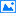图算法面试题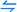排序面试题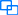线性表面试题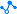二叉树面试题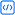高频算法面试题

# 图算法面试题

## 001图相关的术语有哪些？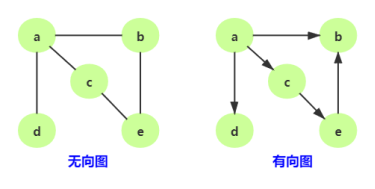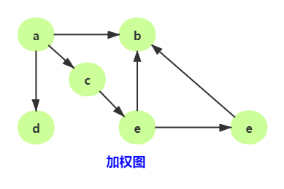1. 通过二维数组，我们可以很快的找到一个顶点和哪些顶点有连线。（比如 A顶点,只需要 遍历第一行即可）
2. 另外，A - A，B - B（也就是顶点到自己的连线），通常使用∞表示。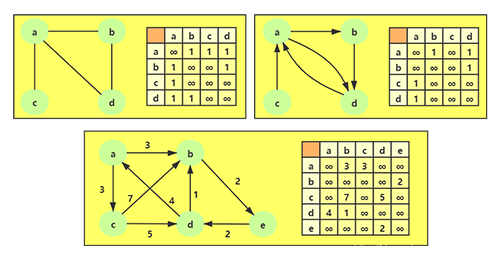## 003邻接矩阵的问题？

1)如果是一个无向图，邻接矩阵展示出来的二维数组，其实是一个对称图。也就是 A -> D 是 1 的时候，对称的位置 D -> 1 一定也是 1。那么这种情况下会造成空间的浪费。
2)邻接矩阵还有一个比较严重的问题就是如果图是一个稀疏图，如果矩阵中将存在大量的∞，这意味着我们浪费了计算机存储空间来表示根本不存在的边。而且即使只有一个边，我们也必须遍历一行来找出这个边，也浪费很多时间。

## 004什么是图的邻接表？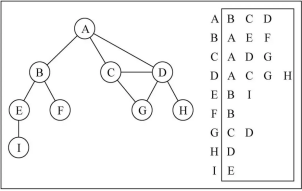## 006如何来封装一个图？

``````public class Node {
public int value;
public int in;
public int out;
public ArrayList<Node> nexts;
public ArrayList<Edge> edges;

public Node(int value) {
this.value = value;
in = 0;
out = 0;
nexts = new ArrayList<>();
edges = new ArrayList<>();
}
}``````

``````public class Edge {
public int weight;
public Node from;
public Node to;

public Edge(int weight, Node from, Node to) {
this.weight = weight;
this.from = from;
this.to = to;
}
}``````

``````public class Graph {
public HashMap<Integer, Node> nodes;
public HashSet<Edge> edges;
public Graph() {
nodes = new HashMap<>();
edges = new HashSet<>();
}
}``````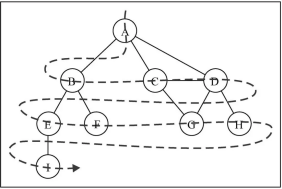## 008宽度优先遍历如何实现？

1. 首先准备一个队列queue和一个辅助set集合
2. 将指定的第一个顶点入队queue,并添加到set集合里面
3. 队列里面的一个顶点出队并输出该顶点的值
4. 遍历该顶点的相邻顶点，如果set集合里面不包含相邻顶点cur，则将cur入队并加入到set集合里面
5. 【3,4】步骤周而复始一次迭代。

``````//从node出发，进行宽度优先遍历
public static void bfs(Node node) {
if (node == null) {
return;
}
HashSet<Node> set = new HashSet<>();
while (!queue.isEmpty()) {
Node cur = queue.poll();
System.out.println(cur.value);
for (Node next : cur.nexts) {
if (!set.contains(next)) {
}
}
}
}``````

## 009什么叫深度优先搜索(DFS，Depth First Search)？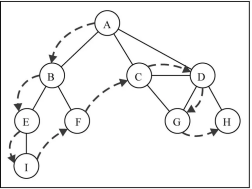## 010深度遍历的具体实现？

1. 首先准备一个栈stack和一个辅助set集合
2. 将指定的第一个顶点入栈stack,并添加到set集合里面，然后输出该顶点的值
3. 栈里面的一个顶点出栈，保存出栈的顶点cur,
4. 遍历该顶点cur的相邻顶点nexts，如果set集合里面不包含相邻顶点cur，则将cur和相邻节点next都入栈并将next节点加入到set集合里面，输出相邻顶点的值，跳出循环。
5. 【3,4】步骤周而复始一次迭代。

``````public static void dfs(Node node) {
if (node == null) {
return;
}
Stack<Node> stack = new Stack<>();
HashSet<Node> set = new HashSet<>();
System.out.println(node.value);
while (!stack.isEmpty()) {
Node cur = stack.pop();
for (Node next : cur.nexts) {
if (!set.contains(next)) {
stack.push(cur);
stack.push(next);
System.out.println(next.value);
break;
}
}
}
}
``````

## 011什么是图的拓扑排序？

1.找一个入度为零（不需其他关卡通关就能解锁的）的端点，如果有多个，则从编号小的开始找；
2.将该端点的编号输出；
3.将该端点删除，同时将所有由该点出发的有向边删除；
4.循环进行 2 和 3 ，直到图中的图中所有点的入度都为零；
5.拓扑排序结束；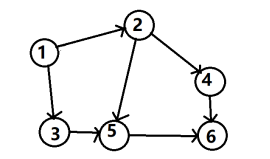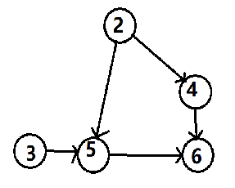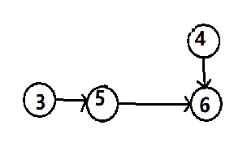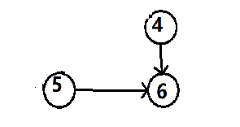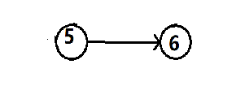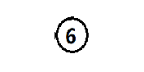## 012图的拓扑排序如何实现？

1. 首先准备一个inMap,其中key为节点node,value为该节点剩余的入度数。然后准备一个队列zeroInQueue
2. 遍历图graph，将图graph中node和以及节点对应的入度数in存储到inMap里面,如果该节点的入度数in为0则将该节点入队zeroInQueue
3. 将队列zeroInQueue的节点出队，保存该节点为cur,把cur存储到集合result里面，遍历当前节点cur的相邻节点，将相邻节点的入度数减1，如果相邻节点的入度数为0则将该节点入队zeroInQueue
4. 【3】步骤周而复始一次迭代。

``````// directed graph and no loop
public static List<Node> sortedTopology(Graph graph) {
// key：某一个node
// value：剩余的入度
HashMap<Node, Integer> inMap = new HashMap<>();
// 入度为0的点，才能进这个队列
for (Node node : graph.nodes.values()) {
inMap.put(node, node.in);
if (node.in == 0) {
}
}
// 拓扑排序的结果，依次加入result
List<Node> result = new ArrayList<>();
while (!zeroInQueue.isEmpty()) {
Node cur = zeroInQueue.poll();
for (Node next : cur.nexts) {
inMap.put(next, inMap.get(next) - 1);
if (inMap.get(next) == 0) {
}
}
}
return result;
}``````

## 014什么是kruskal算法？

Kruskal（克鲁斯卡尔算法）算法是一种用来查找最小生成树的算法。Kruskal算法是基于贪心的思想得到的。首先我们把所有的边按照权值先从小到大排列，接着按照顺序选取每条边，如果这条边的两个端点不属于同一集合，那么就将它们合并，直到所有的点都属于同一个集合为止。换而言之，Kruskal算法就是基于并查集的贪心算法。

## 015kruskal算法具体实现？

``````public static class MySets {
public HashMap<Node, List<Node>> setMap;
public MySets(List<Node> nodes) {
for (Node cur : nodes) {
List<Node> set = new ArrayList<Node>();
setMap.put(cur, set);
}
}
public boolean isSameSet(Node from, Node to) {
List<Node> fromSet = setMap.get(from);
List<Node> toSet = setMap.get(to);

return fromSet == toSet;
}
public void union(Node from, Node to) {
List<Node> fromSet = setMap.get(from);
List<Node> toSet = setMap.get(to);

for (Node toNode : toSet) {
setMap.put(toNode, fromSet);
}
}
}
``````

## 016什么是prim算法？

prim算法 (普里姆算法)我们先初始定义一个顶点u，然后在相邻的所有边中找到最小权值的边 e1，将顶点u链接到初始点c之外的顶点v,之后将顶点v放到c中，并且一直重复知道完成。

## 017prim算法的具体实现？

1. 准备一个边的小根堆队列priorityQueue、顶点的集合set和要挑选的边的集合result
2. 遍历图的顶点nodes，将随机的一个顶点node放到集合set里面，将顶点node所相连所相连的边入队到小根堆队列priorityQueue。
3. 弹出队列里面最小权重的边，判断这个边的to节点的是不是在set集合里面，如果不在，则将to节点放到set里面，并把这条边存到result里面。再将to顶点所相连所相连的边入队到priorityQueue。周而复始依次迭代。
4. 3步骤之后break，跳出循环。

``````public static Set<Edge> primMST(Graph graph) {
// 解锁的边进入小根堆
PriorityQueue<Edge> priorityQueue = new PriorityQueue<>(new EdgeComparator());
HashSet<Node> set = new HashSet<>();
Set<Edge> result = new HashSet<>(); // 依次挑选的的边在result里
for (Node node : graph.nodes.values()) { // 随便挑了一个点
// node 是开始点
if (!set.contains(node)) {
for (Edge edge : node.edges) { // 由一个点，解锁所有相连的边
}
while (!priorityQueue.isEmpty()) {
Edge edge = priorityQueue.poll(); // 弹出解锁的边中，最小的边
Node toNode = edge.to; // 可能的一个新的点
if (!set.contains(toNode)) { // 不含有的时候，就是新的点
for (Edge nextEdge : toNode.edges) {